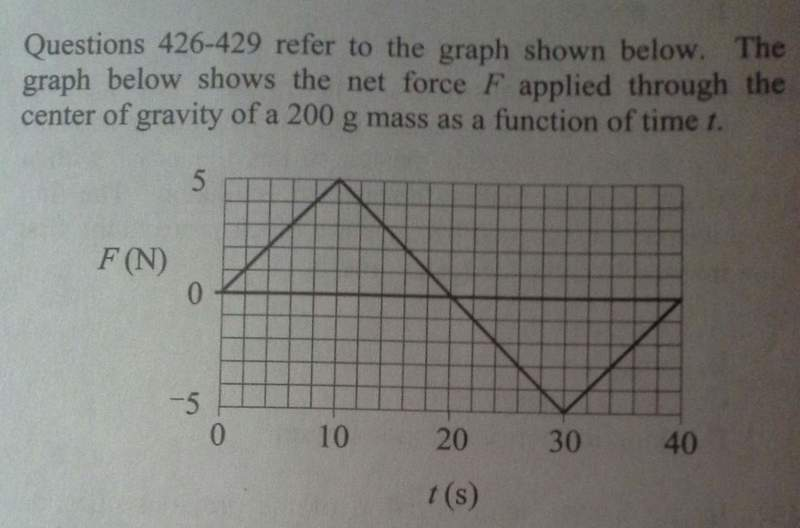# Interpretation of an impulse graph

lovelyrwwrWhich of the following is true if the mass is at rest at t=0 ?
A. Velocity of the mass is decreasing btwn 10 and 20 seconds.
B. The accel. of the mass is constant between 0 and 10 seconds
C. At 40 seconds the mass is stationary.
D. The distance trsveled by the mass bten 0 and 10 seconds is equal to that traveled btwn 10 and 20 seconds.

The answer is C. I understand why I can rule out answer B. But I do not see why A and D are not true.

From the graph, I see that force decreases during 10 < t < 20 seconds. Thus accel gets smaller but is still positive, which means that velocity should still be increasing. However what I do not understand is what the inflection point at t=10 (and t=30 for that matter) represents...I mean between 0-20 seconds, velocity is always increasing (according to the solution, but then what does this inflection point at t=10 seconds represent?

This problem is driving me insane, plz help! :)

## Answers and Replies

Homework Helper
View attachment 62919
Which of the following is true if the mass is at rest at t=0 ?
A. Velocity of the mass is decreasing btwn 10 and 20 seconds.
B. The accel. of the mass is constant between 0 and 10 seconds
C. At 40 seconds the mass is stationary.
D. The distance trsveled by the mass bten 0 and 10 seconds is equal to that traveled btwn 10 and 20 seconds.

The answer is C. I understand why I can rule out answer B. But I do not see why A and D are not true.

From the graph, I see that force decreases during 10 < t < 20 seconds. Thus accel gets smaller but is still positive, which means that velocity should still be increasing. However what I do not understand is what the inflection point at t=10 (and t=30 for that matter) represents...I mean between 0-20 seconds, velocity is always increasing (according to the solution, but then what does this inflection point at t=10 seconds represent?

This problem is driving me insane, plz help! :)

From what you've just said, you can already rule out A. The points at x=10 and x=30 are just the points of maximum acceleration and deceleration respectively. Now you just have to rule out D. I'm not sure what's driving you insane.

lovelyrwwr
So btwn t = 0 and 10 seconds the mass is accelerating, right? And from 10 to 20 sec it is still accelerating but acceleration is declining...so shouldnt answer A be true?

Wow im such a dope! Thanks for all of ur help!!!)))